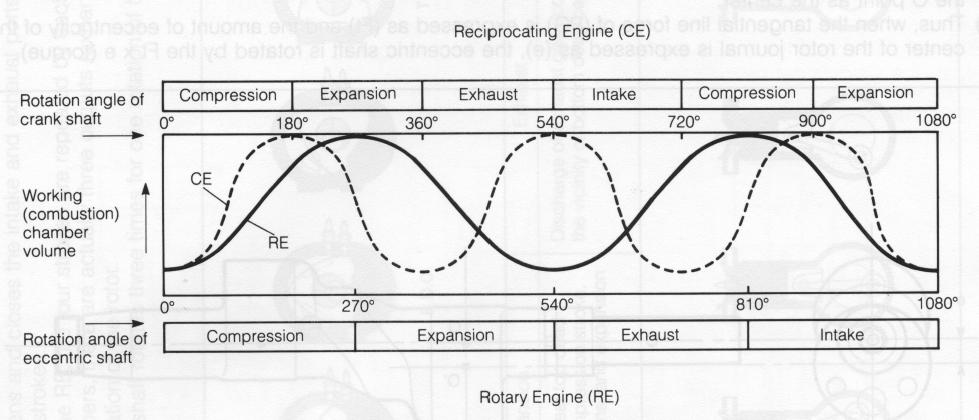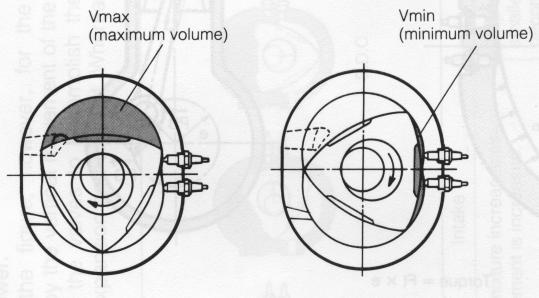PROCESS TIME

As shown in the figure, for the operation of each stroke the rotational angle of the RE eccentric shaft is 270°, whereas the rotational angle of the CE crankshaft is 180°.

The advance time is longer for the RE, with they result that more complete air intake is provided at high rpm, thus providing high torque.

Fig. 3-3 Process time

DISPLACEMENT AND COMPRESSION RATIO

For the RE, there are three working chambers for one rotor, but the displacment is figured as the volume which results from the multiplication of the volume of one chamber by the number of rotors. Thus, displacement equals: single-chamber volume x 2 (number of rotors). The single-chamber volume is the volume which results from subtracting Vmin from Vmax.

Displacement = (single chamber volume) x (number of rotors) Single chamber volume = (Vmax) - (Vmin) Compression ratio = (VmaxNmin) ; 1

Mazda REs

10A ... 491 cc (30 cu. in.) x 2 12A ... 573 cc (35 cu. in.) x 2 13B ... 654 cc (40 cu. in.) x 2

The compression ratio is the ratio of Vmax and Vmin.

10A...9.4:1 12A...9.4:1 13B...9.4:1

Fig. 3-4 Displacement and compression ratio